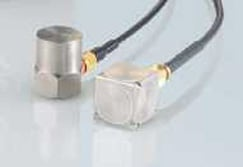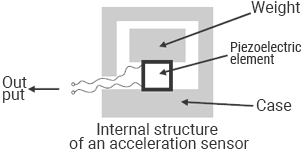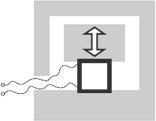# Basics of Acceleration Measurement

There are various types of acceleration sensors to match various measurement targets. This section explains the types and operating principles of these acceleration sensors.

## Types of acceleration sensorsAcceleration sensors are designed to measure the acceleration (rate of change in speed) of an object.
Acceleration sensors are generally classified into the following four types.

1. Piezoelectric-type acceleration sensors
2. Servo-type acceleration sensors
3. Strain gauge-type acceleration sensors
4. Semiconductor-type acceleration sensors

Among these sensors, piezoelectric-type acceleration sensors are regarded as the most common type due to their compact size, wide range, high sensitivity, etc.

### Piezoelectric effect

The piezoelectric effect refers to a phenomenon where polarization, which generates voltage, occurs through application of force to a piezoelectric body (on the contrary, a phenomenon where force is generated through application of voltage to a piezoelectric body is called the “inverse piezoelectric effect”). Piezoelectric-type acceleration sensors use the piezoelectric effect to measure the acceleration.
Typical piezoelectric bodies include crystal (SiO2) and Rochelle salt (KNaC4H4O6).

## Principles of acceleration sensorsAn acceleration sensor uses its internal piezoelectric element to measure the motion of an object.A piezoelectric element generates electric charges when it expands or contracts. The acceleration is measured using the output of electric charges generated by this expansion/contraction of the element.
The piezoelectric element expands or contracts according to the upward/downward movement of the weight and generates electric charges in response to this change.

INDEX